tech@7universum.com
+7 (499) 938-98-57

# Heat-conducting properties of polymeric materials

Теплопроводные свойства полимерных материалов
Цитировать:
Yusupova R.K., Umarov A.A., Mirzaxonova G.G. Heat-conducting properties of polymeric materials // Universum: технические науки : электрон. научн. журн. 2021. 2(83). URL: https://7universum.com/ru/tech/archive/item/11301 (дата обращения: 26.09.2021).
Прочитать статью:

ABSTRACT

In the given paper it is reduced data on the common of properties of heat conduction of solids, in particular it is displayed their special difference in the course of heat transfer of uncrystalline and crystalline polymers

АННОТАЦИЯ

В данной статье приведены сведения об общих свойств теплопроводности твердых тел, в частности показано их особые отличия в процессе теплопередачи аморфных и кристаллических полимеров.

Keywords: flexible, a crystallite, a circuit, polymer, homogeneous, elasticity

Ключевые слова: гибкий, кристаллит, цепь, полимер, однородный, упругость

Introduction. When thermal conductivity is studied for solids, the equation of temperature, that is, the state resulting from the transfer of heat from the heated part to the rest, is understood. If a thin long rod is obtained, the temperature at the ends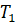and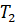, if  the length of the rod is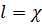obtained, then the temperature gradient of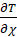appears on the rod. This gradient creates a heat flow along the stem.

Fure's law of thermal conductivity is expressed as follows: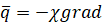(1)

Where,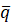is the heat flux density vector (numerically, the amount of energy passing through the sample cross section per unit time)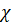- heat transfer coefficient (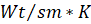)

Thermal conductivity reflects the energy transfer in itself. As with any other migration process, thermal conductivity has a relaxing nature. This is because when the temperature in an element is changed, the temperature gradient at the changed location creates a heat flux until it drops to zero. If an artificially constant temperature gradient is maintained, a constant (steady) heat flux will occur over time.

All solids can be divided into three major groups in terms of heat transfer:

1. Mirrors, polymeric materials (dielectrics);

2. Metals;

3. Composite polymer materials;

Heat transfer in polymeric materials is due to the vibration of crystal lattices, electrons in metals, and in composite polymeric materials due to crystal lattices and electrons.

Let us considerthe bonds of a single polymer in the crystalline and amorphous state (Fig. 1).

The main element in the structure of crystalline polymers are crystallites. It is known that polymers are divided into rigid chain polymers and flexible chain polymers depending on the type of chain. Solid-chain polymers form straight-line chains. Flexible chain polymers, on the other hand, form crystal plates-lamellae, which are folded with a certain regularity during crystallization. The lamellae, in turn, form larger spherolites. As can be seen from the above, crystalline lattices lie at the base of the lamellae and spherolites. If, as a set of harmonic oscillators in a crystal lattice, the normal oscillations are not related to each other and the oscillation amplitude is temperature dependent, no uniform distribution of energy is observed in such a system due to the independence of the individual oscillations and the absence of energy effects.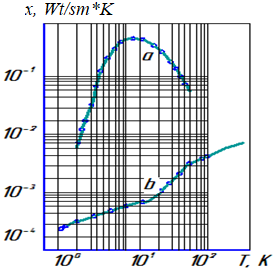Figure 1. Temperature dependence of thermal conductivity

a-crystal sample; b-amorphous specimen

Debay proposed the following formula for determining the wavelength and thermal conductivity of grids :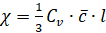(2)

where,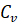- is the heat capacity corresponding to the unit volume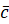- average sound speed- the average length of the path traversed by a free phonon

The duration of the phonon is as follows: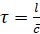(3)

At relatively high temperatures (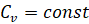), the speed of sound is considered to be independent of the temperature at the Debay approach, since12 is inversely proportional to the temperature. As the temperature decreases,increases and at lower temperatures it becomes equal to the size ofcrystals. If the temperature continues to drop, it will be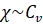. At very low temperatures it is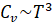, and varies with such regularity depending on the temperature 1. Thus, the thermal conductivity in crystalline polymers decreases with increasing temperature (Figure 1).

The development of a theory that allows the calculation of the thermal conductivity coefficient as a function of temperature is one of the serious problems in solid state physics. Some deviations are allowed in solving this problem, and this is certainly not always the case.

In amorphous polymers, the study of thermal conductivity is complicated by the lack of arrangement of atoms along the translational symmetry. Regarding the structure of amorphous polymers, the famous American scientist A. Tobolsky said: “The structure of amorphous polymers is similar to thin pasta boiled in a pot; "Pasta molecules are in constant chaotic motion, their amplitude and speed depend on temperature" . Therefore, the temperature-dependent change in the thermal conductivity in amorphous polymers differs qualitatively from the bonding of crystalline polymers.

Thus, it can be seen that amorphous polymers have a qualitatively completely different mechanism of thermal conductivity. Formula (2) is used to determine the thermal conductivity of amorphous polymers. The main problem is to determine the average length of the phonon's path. Using the results of a known experiment, it is possible to determine the average length of the path traversed by a phonon in some cases using formula (2). For example, Kittel showed that the average path length of a phonon is equal to the size of the structural unit of an amorphous quartz glass . In fact, the average length of a free-flowing phonon in a room-temperature quartz glass is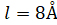, and the size of a silicon tetrahedral cell is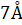. At one time, Clemens made the same point when analyzing thermal conductivity.

Due to the disordered arrangement of atoms in amorphous polymers, the elastic property of the polymer varies from one point to another. If an elastic wave is propagated in such a polymer, the propagation of the wave will be the same as in a non-homogeneous medium. Thus, the speed of phonons also varies from one point to another. This in turn leads to an increase in the thermal conductivity of amorphous polymers with increasing temperature (Figure 1).

In short, in order to increase the thermal conductivity of polymeric materials, it is necessary to include compositions based on powder or fibrous metal, graphite, carbon and other materials with high thermal and electrical conductivity. Conversely, in order to reduce the thermal conductivity, it is advisable to create cellular structures in polymeric materials.

References:

1. Перепечко И.И. Введение в физику полимеров. М.,1978, 310 с.
2. Тобольский А. Свойства и структура полимеров. Пер. с анг. М.,”Химия”, 1964. 322 с.
3. Киттель Ч. Ввведение в физику твердого тела. Изд. 2-е. Пер. с анг. М., Физматгиз, 1963, 696 с.
4. Клеменс П.В. Физика низких температур. Пер. с анг. М., Издатинлит, 1959,  314 с.
5. Батаев А.А. Композиционные материалы. Новосибирск,  Изд. НГТУ,   2002, 384 с.
Информация об авторахSenior teacher  Andijan machine building institute, Uzbekistan, Andijan

старший преподаватель, Андижанский машиностроительный институт, Узбекистан, г. АндижанStudent Andijan machine building institute, Uzbekistan, Andijan

студент, Андижанский машиностроительный институт, Узбекистан, г. АндижанStudent Andijan machine building institute, Uzbekistan, Andijan

студент, Андижанский машиностроительный институт, Узбекистан, г. Андижан

Журнал зарегистрирован Федеральной службой по надзору в сфере связи, информационных технологий и массовых коммуникаций (Роскомнадзор), регистрационный номер ЭЛ №ФС77-54434 от 17.06.2013
Учредитель журнала - ООО «МЦНО»
Главный редактор - Ахметов Сайранбек Махсутович.
Top